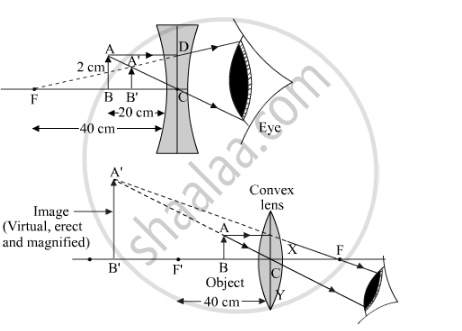Share

# (A) Find the Position and Size of the Virtual Image Formed When an Object 2 Cm Tall is Placed 20 Cm From: (I) a Diverging Lens of Focal Length 40 Cm. (Ii) a Converging Lens of Focal Length 40 Cm. (B) Draw Labelled Ray Diagrams to Show the Formation of Images in Case (I) and (Ii) Above (The Diagrams May Not Be According to Scale). - CBSE Class 10 - Science

ConceptRefraction of Light Image Formation by Lenses - Concave Lens

#### Question

(a) Find the position and size of the virtual image formed when an object 2 cm tall is placed 20 cm from:
(i) a diverging lens of focal length 40 cm.
(ii) a converging lens of focal length 40 cm.
(b) Draw labelled ray diagrams to show the formation of images in case (i) and (ii) above (The diagrams may not be according to scale).

#### Solution

(a) Object height (h) = 2 cm
Image height (h') =?
Object distance (u) = -20 cm (sign convention)
(i) Focal length (f) = -40 cm (sign convention)
Image distance (v) = ?

Applying the lens formula:

1/v-1/u=1/f

1/v-1/-20=1/-40

1/v+1/20=-1/40

1/v=-1/40-1/20

1/v=(-1-2)/40

1/vv=-3/40

∴ v=-40/3=-13.33 cm

Negative sign shows that the image formed is virtual and erect.

Now, magnification m =

v/u=h^'/h

h^1=v/uxh

h^i=(-13.33)/-20x2=1.33cm

Height of the image is 1.33 cm.

(ii) Focal length (f) = 40 cm (sign convention)
Image distance (v) = ?
Applying the lens formula:

1/v-1/u=1/f

1/v-1/-20=1/40

1/v+1/20=1/40

1/v=1/40-1/20

1/v=(1-2)/40

1/v=(-1)/40

∴v=-40 cm

Negative sign shows that the image formed is virtual and erect.

Now, magnification =

v/u=h^'/h

h^'=v/uxh

h'=-40/-20X2=4cmIs there an error in this question or solution?

#### APPEARS IN

Solution (A) Find the Position and Size of the Virtual Image Formed When an Object 2 Cm Tall is Placed 20 Cm From: (I) a Diverging Lens of Focal Length 40 Cm. (Ii) a Converging Lens of Focal Length 40 Cm. (B) Draw Labelled Ray Diagrams to Show the Formation of Images in Case (I) and (Ii) Above (The Diagrams May Not Be According to Scale). Concept: Refraction of Light - Image Formation by Lenses - Concave Lens.
S